# Arrays Thomas Schwarz SJ Example Python lists are

• Slides: 33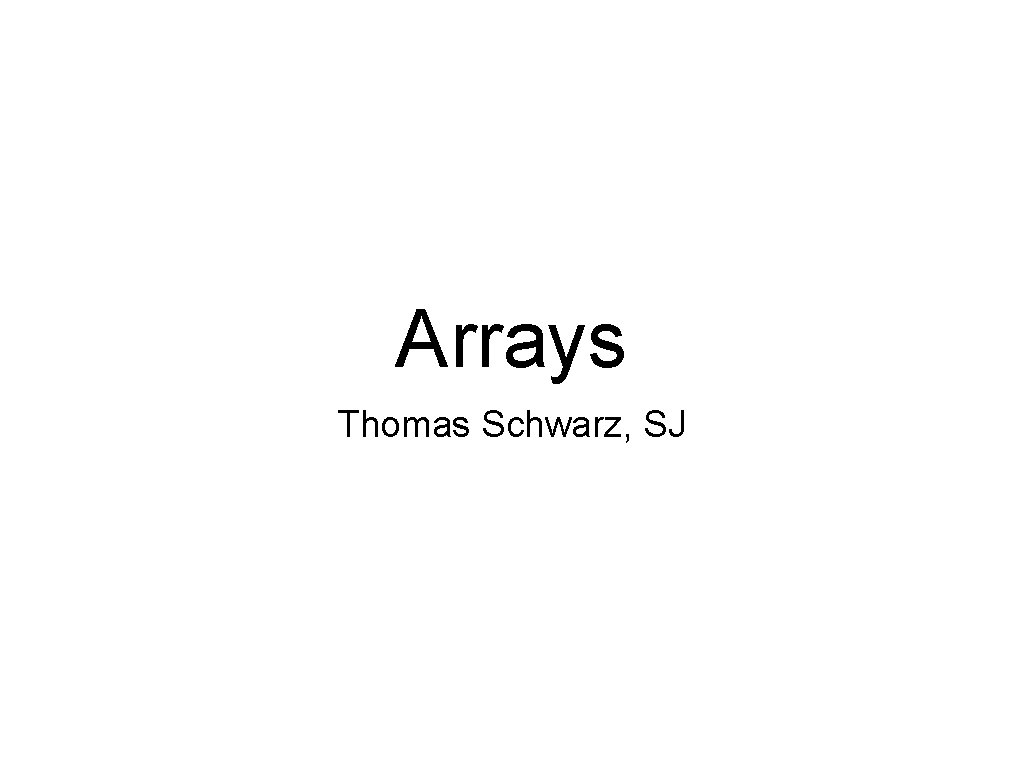Arrays Thomas Schwarz, SJExample • • Python lists are internally organized as arrays of object pointers Python sets are internally organized as hash-tables Experiment: • Create a set and a list with the same 100, 000 random elements • Then search for the first 100, 000 elements in the set and the list Result: List is much slower (timing in sec) • • array: 126. 1766300201416 set: 0. 00663900375366210 (20, 000 times faster)Array ADT • Classic array: • • • Accessed by index Python implementation: • • Collection of objects of the same type Python has lists, which internally are organized as pointers to objects Num. Py has a better performing arrayNum. Py Fundamentals • Numpy is a module for faster vector processing with numerous other routines • Scipy is a more extensive module that also includes many other functionalities such as machine learning and statisticsNum. Py Fundamentals • Install numpy with pip 3 • If pip 3 does not show up in your terminal window, then you did not set the Python path correctlyNum. Py Fundamentals • Why Numpy? • Remember that Python does not limit lists to just elements of a single class • If we have a large list and we want to add a number to all of the elements, then Python will asks for each element: • • What is the type of the element Does the type support the + operation Look up the code for the + and execute This is slowNum. Py Fundamentals • Why Numpy? • Primary feature of Numpy are arrays: • List like structure where all the elements have the same type • • • Usually a floating point type Can calculate with arrays much faster than with list Implemented in C / Java for Cython or Jython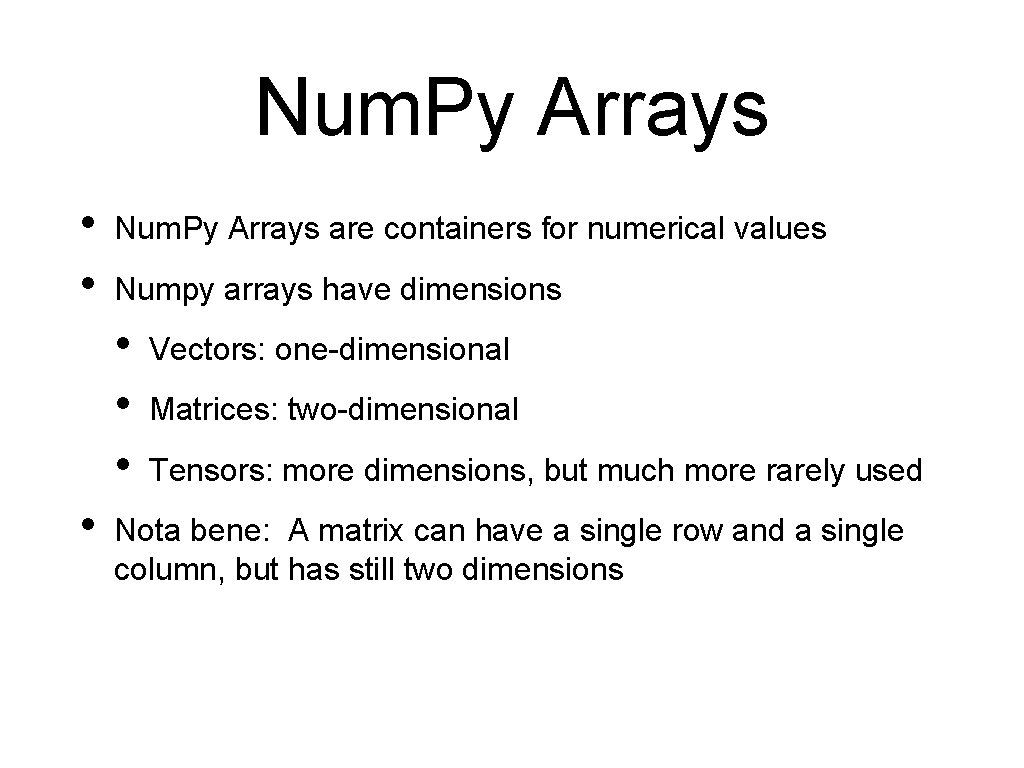Num. Py Arrays • • Num. Py Arrays are containers for numerical values Numpy arrays have dimensions • • Vectors: one-dimensional Matrices: two-dimensional Tensors: more dimensions, but much more rarely used Nota bene: A matrix can have a single row and a single column, but has still two dimensions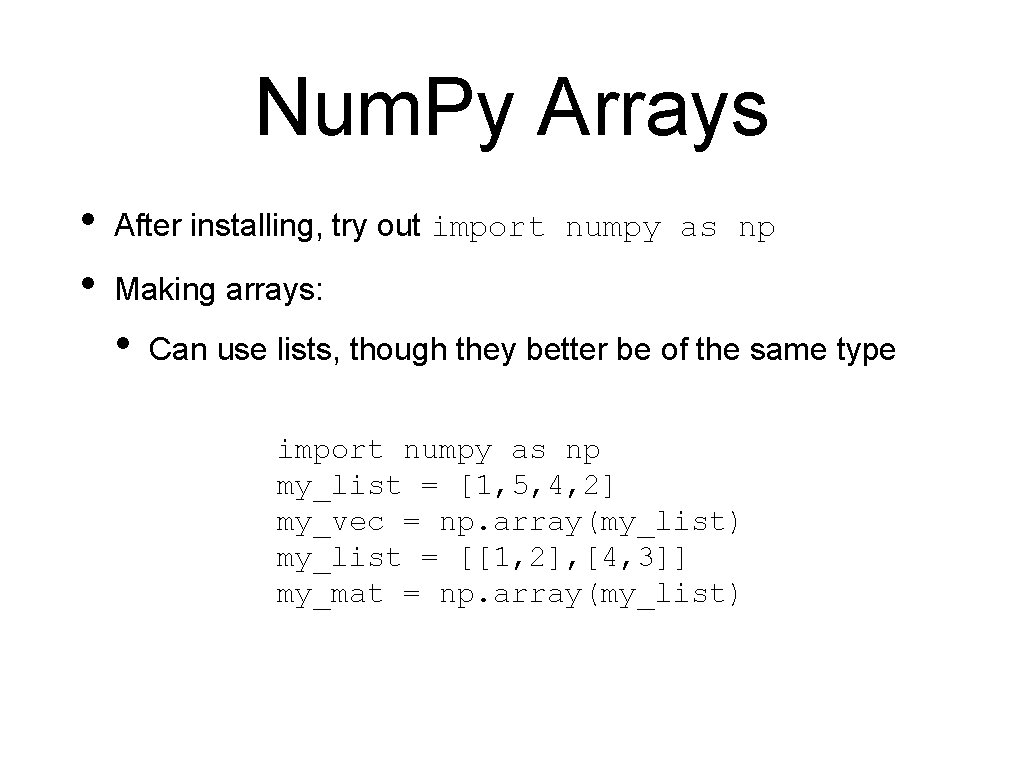Num. Py Arrays • • After installing, try out import numpy as np Making arrays: • Can use lists, though they better be of the same type import numpy as np my_list = [1, 5, 4, 2] my_vec = np. array(my_list) my_list = [[1, 2], [4, 3]] my_mat = np. array(my_list)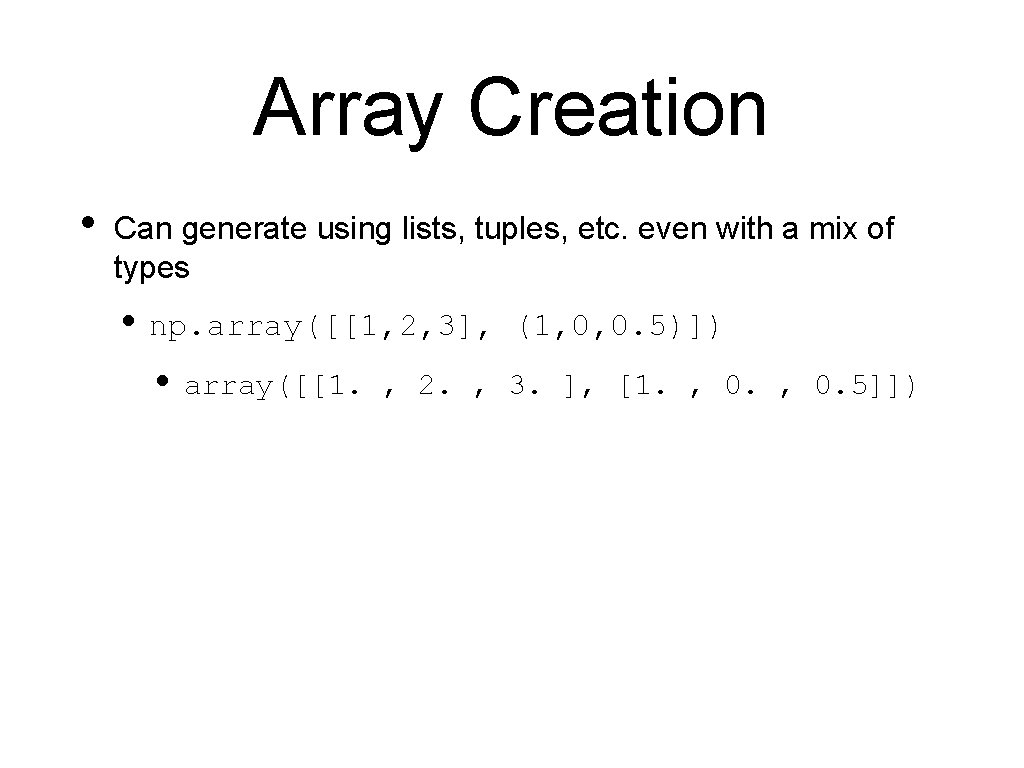Array Creation • Can generate using lists, tuples, etc. even with a mix of types • np. array([[1, 2, 3], • array([[1. (1, 0, 0. 5)]) , 2. , 3. ], [1. , 0. 5]])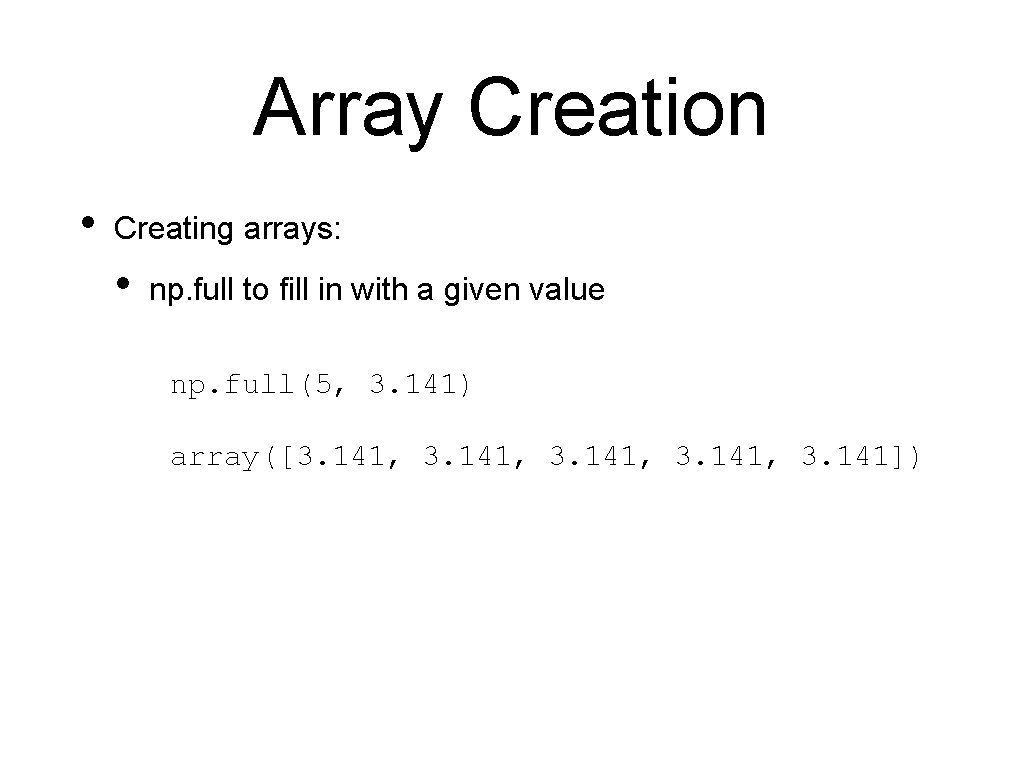Array Creation • Creating arrays: • np. full to fill in with a given value np. full(5, 3. 141) array([3. 141, 3. 141])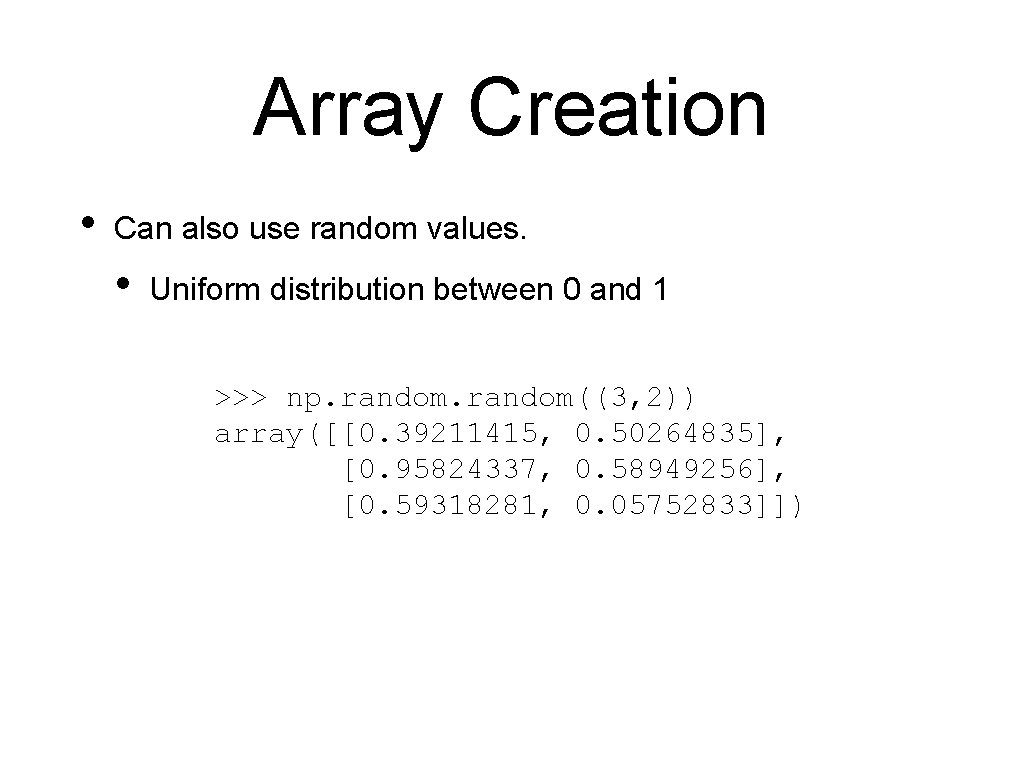Array Creation • Can also use random values. • Uniform distribution between 0 and 1 >>> np. random((3, 2)) array([[0. 39211415, 0. 50264835], [0. 95824337, 0. 58949256], [0. 59318281, 0. 05752833]])Array Creation • Or random integers >>> np. random. randint(0, 20, (2, 4)) array([[ 5, [19, 7, 7, 2, 10], 1, 10]])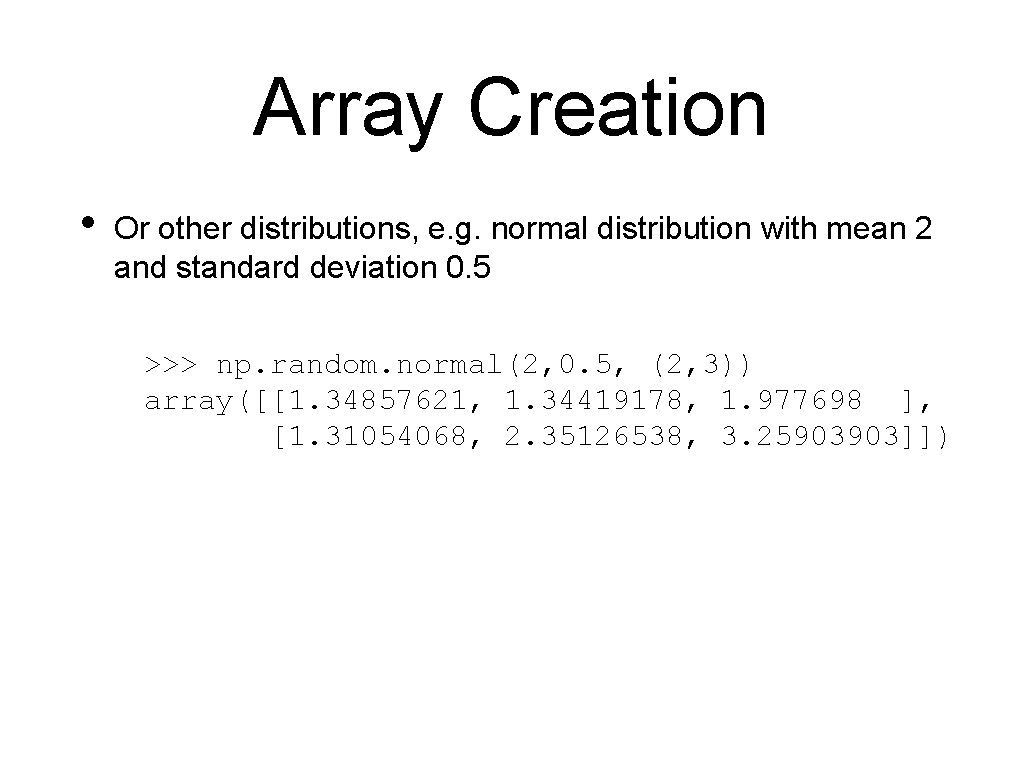Array Creation • Or other distributions, e. g. normal distribution with mean 2 and standard deviation 0. 5 >>> np. random. normal(2, 0. 5, (2, 3)) array([[1. 34857621, 1. 34419178, 1. 977698 ], [1. 31054068, 2. 35126538, 3. 25903903]])Array Creation • fromfunction >>> x = np. fromfunction(lambda i, j: (i**2+j**2)//2, (4, >>> x. astype(int) array([[ [ 0, 0, 2, 4, >>> x. shape (4, 5) 0, 1, 2, 5, 2, 2, 4, 6, 4, 8], 5, 8], 6, 10], 9, 12]])Array Creation • Creating from download / file • We use urllib. request module • If you are on Mac, you need to have Python certificates installed • Go to your Python installation in Applications and click on "Install Certificates command"Array Creation • Use urllib. request. urlretrieve with website and file name • Remember: A file will be created, but the directory needs to exist import urllib. request. urlretrieve( url = "https: //ndownloader. figshare. com/files/125656 filename = "avg-monthly-precip. txt" ) • • This is a text file, with one numerical value per line Then create the numpy array using avgmp = np. loadtxt(fname = 'avg-monthly-precip. txt') print(avgmp)Array Creation • • Example: Get an account at openweathermap. org/appid Install requests and import json • Use the openweathermap. org api to request data on a certain town • Result is send as a JSON dictionaryArray Creation import numpy as np import requests import json mumbai=json. loads(requests. get('http: //api. openweathermap. or vasai = json. loads(requests. get('http: //api. openweathermap. o navi_mumbai = json. loads(requests. get('http: //api. openweathe chalco = json. loads(requests. get('http: //api. openweathermap. milwaukee = json. loads(requests. get('http: //api. openweatherm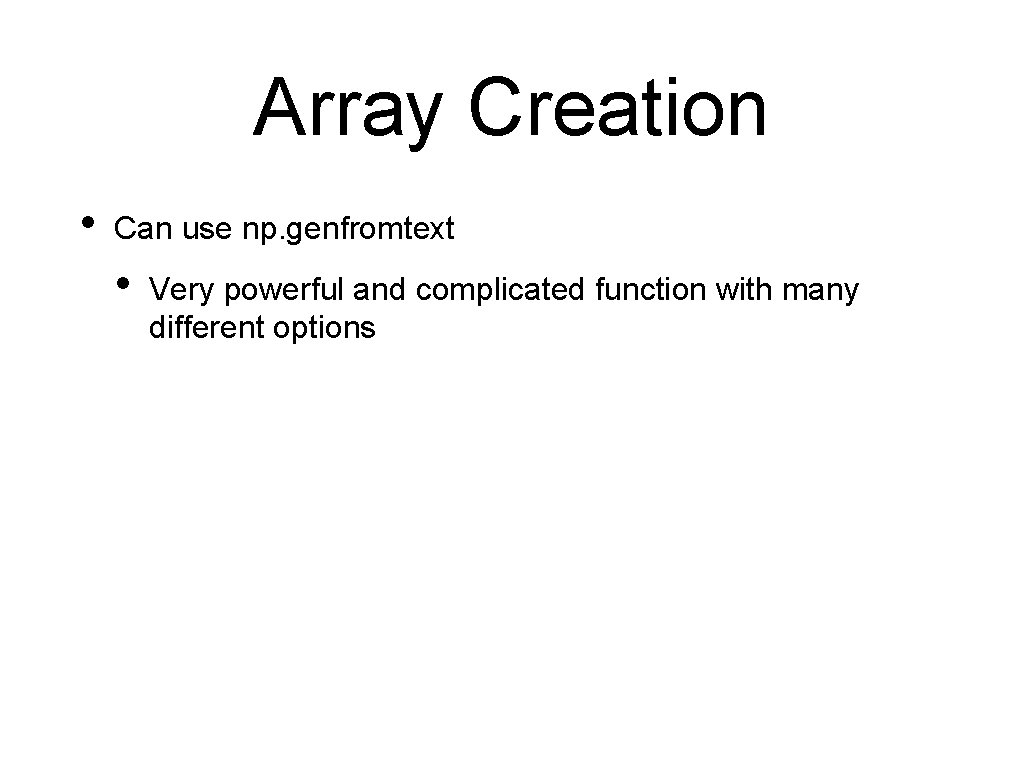Array Creation • Can use np. genfromtext • Very powerful and complicated function with many different optionsArray Creation • Example converters = {5: lambda x: int(0 if 'Iris-setosa' else 1 i my_array = np. genfromtxt('. . /Classes 2/Iris. csv', usecols=(1, 2, 3, 4, 5), dtype =[float, floa delimiter = ', ', converters = converters, skip_header=1) • • • Need a source (the iris file) Can specify the columns we need Give the dtype U 20 -unicode string, S 20 -byte string Delimiter Skipheader, skipfooter converters to deal with encodingArray Creation • • This is an array of 150 tuples Use comprehension to convert to a two-dimensional array m = np. array( [ [row, row, row, row, row] for row in my_array ])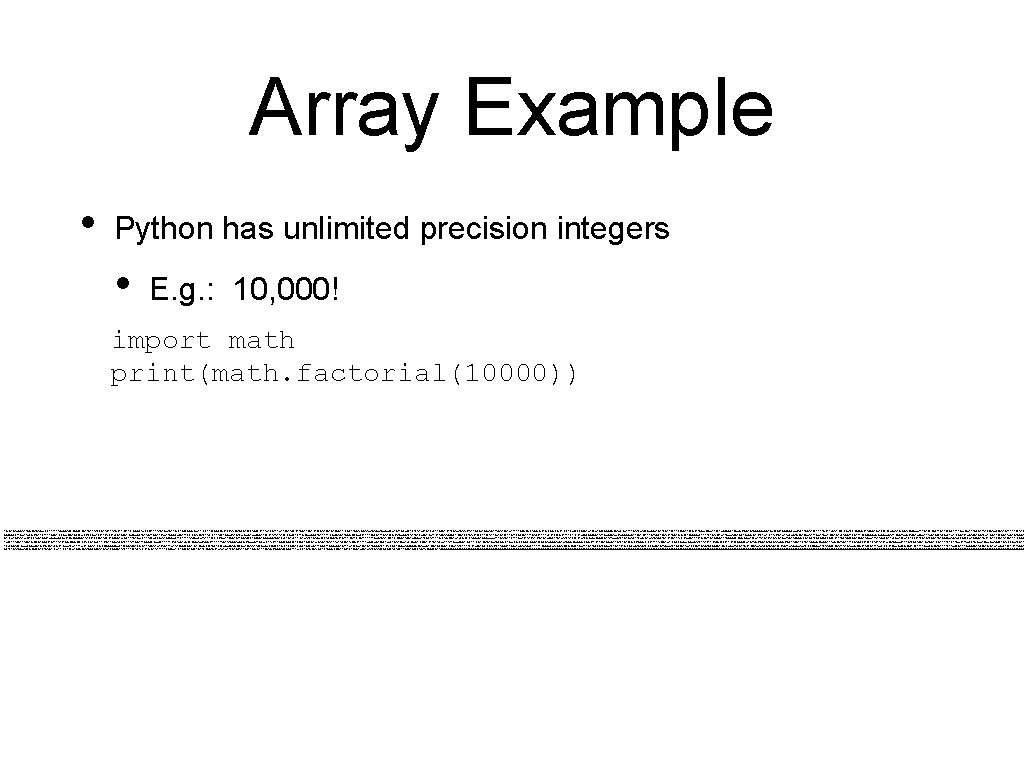Array Example • Python has unlimited precision integers • E. g. : 10, 000! import math print(math. factorial(10000)) 284625968091705451890641321211986889014805140170279923079417999427441134000376444377299078675778477581588406214231752883004233994015351873905242116138271617481982419982759241828925978789812425312059465996259867065601615720360323979263287367170557419759620994797203461536981198970926112775004841988454104755446424421365733030767036288258035489674611170973695786036701910715127305872810411586405612811653853259684258259955846881464304255898366493170592517172042765974074461334000541940524623034368691540594040662278282483715120383221786446271838229238996389928272218797 550883312867263841871327778087444664387535264473356244113944762878097465068395298210817496795883645227334469487379347179071006497823646601668057203429792920744682232284866583952221144685957285840386337727803022759153049786587391951365024627419589908837438733159428737202977062020712021303857217593321116241333042277374241635355358797706530964768588607730143277829032889479581840437885856777293209447677866935753746004814237674119418267163687048105691115621561435751629052735122435008060465366891745819654948260861226075029306276147881326895528073614902252581968281505 524162039926441331651884768606830642004858557924473340290142588876403712518642229016333691585063273727199596362912783344786218887871009533753551054688980236378263714926913289564339440899470121452134572117715657591451734895195016800621353927175419843876163543479806920886666227099512371706241924914282576453125769939735341673046864585181979668232015693792684926999983992413571941496882273704022820805171808003400480615261792013978945186295290558440703738300533552421153903385185829366779190610116306233673144419202893857201855569596330833615450290424822309297087124788 765317950719082045251004478212913185440548144941518671142071036938911291250127508534663377177493760165434546963900427111298292550968304206657253642794722000208353138837087816499571897176293387948542712768826520037663259245616148687448974715193662192756658524621144574070106753804275641844408348052038382650526016985840600847884224218878569278977518104428054744272294551674203356864606099779731249504333214252050536757904995207835976504153790011325795360406551726548790221735954441511394292316489506631778130390574620824491719213118641296337046614064569001789423567387 236755982466625060875428487610414566136222764240591430445558085631818093523040779389161490211629240051507491406844320323036560995487862099919430656445533254713555736531851601170032155069078771675206288152788589714941032098698408304896652435103050244467993177914765910342894912905412036160169567122214080636940594030455218621287993309285623102241844636528909744464015198662318388196244482259078358591404368619301904145896269387890703498216986869693444808621399053459179282665430479820721963413475564652548314377115667845907779719651077246800029358154626764631022427900 83231897086903040030132595147677423751615884091583805915167350451913117819394342848292227230406142258207802782914807042676162930253922832108491775998420059510531216473181840949313980044407284732590260916973099815385393903128087882390294800157900800000000000000000000000000000000000000000000000000000000000000000000000000000000000000000000000000000000000000000000000000000000000000000000000000000000000000000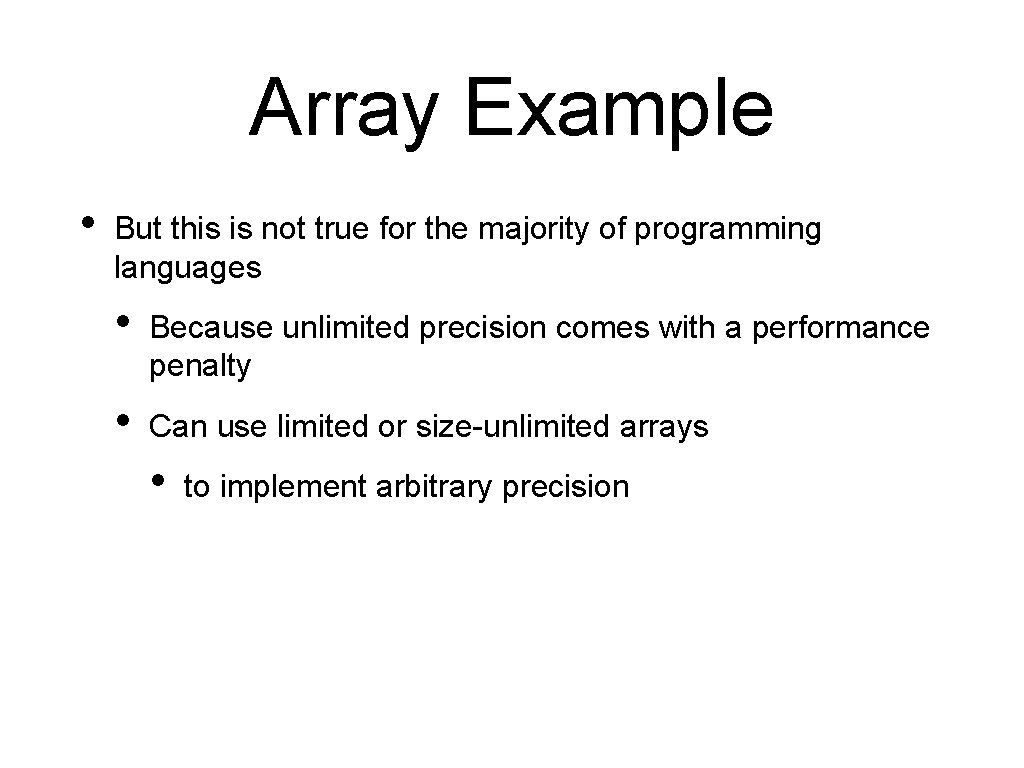Array Example • But this is not true for the majority of programming languages • Because unlimited precision comes with a performance penalty • Can use limited or size-unlimited arrays • to implement arbitrary precisionArray Example • Example: • Let's use Numpy arrays • Numpy arrays have a type • We use np. uint 8 • • 8 -bit unsigned integers 0, 1, . . . , 254, 255 Normal operations roll over • E. g. 250+10=4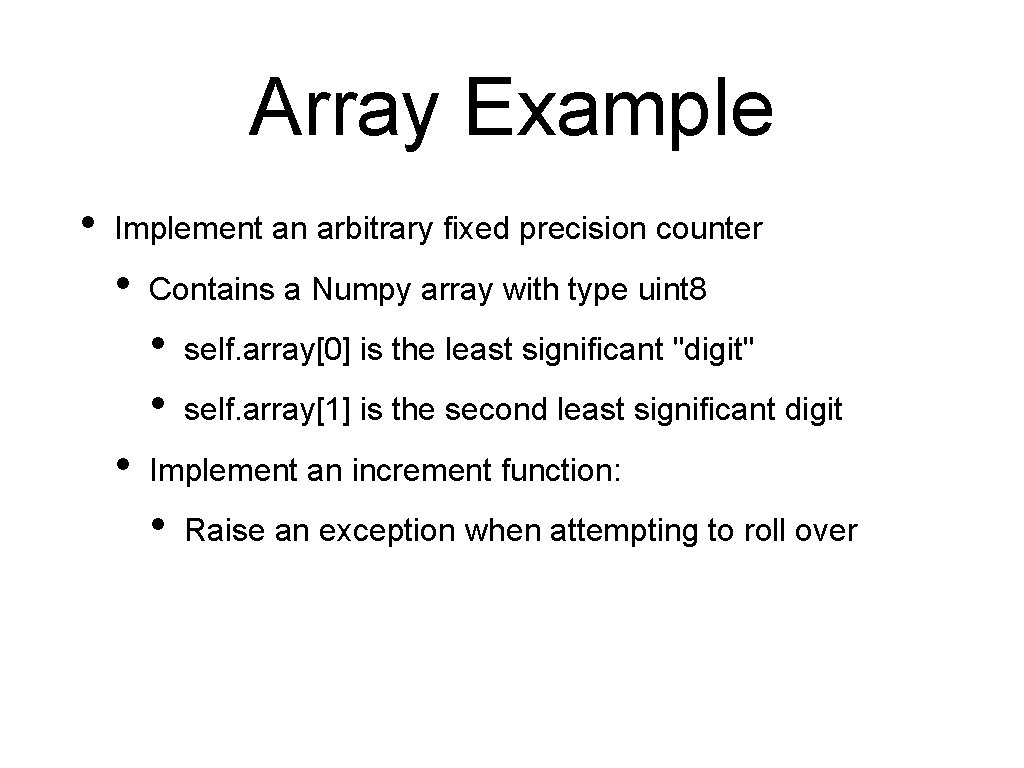Array Example • Implement an arbitrary fixed precision counter • Contains a Numpy array with type uint 8 • • • self. array is the least significant "digit" self. array is the second least significant digit Implement an increment function: • Raise an exception when attempting to roll over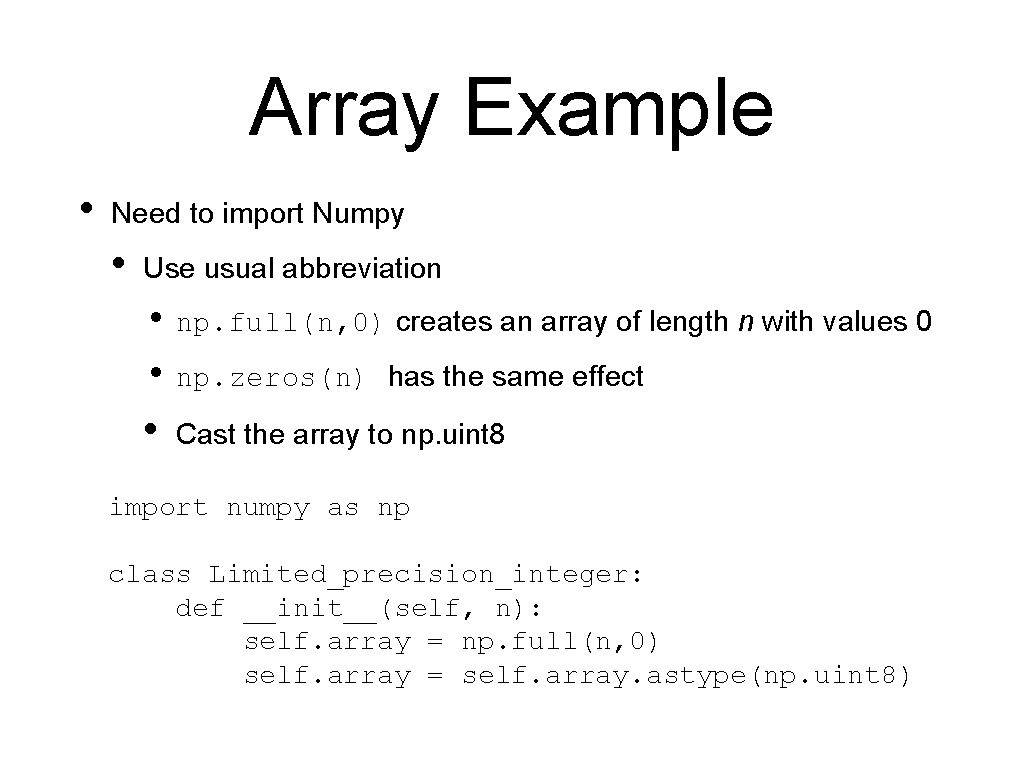Array Example • Need to import Numpy • Use usual abbreviation • np. full(n, 0) creates an array of length n with values 0 • np. zeros(n) has the same effect • Cast the array to np. uint 8 import numpy as np class Limited_precision_integer: def __init__(self, n): self. array = np. full(n, 0) self. array = self. array. astype(np. uint 8)Array Example • Implementing the increment function • We add one to the current "digit" • • We stop if the increment does not roll over For the last digit: • If we are about to roll-over • Raise a Value. ErrorArray Example raise Value. Error('LPI rollover to zero') Value. Error: LPI rollover to zeroArray Example def incr(self): for i in range(len(self. array)-1): self. array[i] += 1 if self. array[i] != 0: return if self. array[-1] < 255: self. array[-1]+=1 else: raise Value. Error('LPI rollover to zero')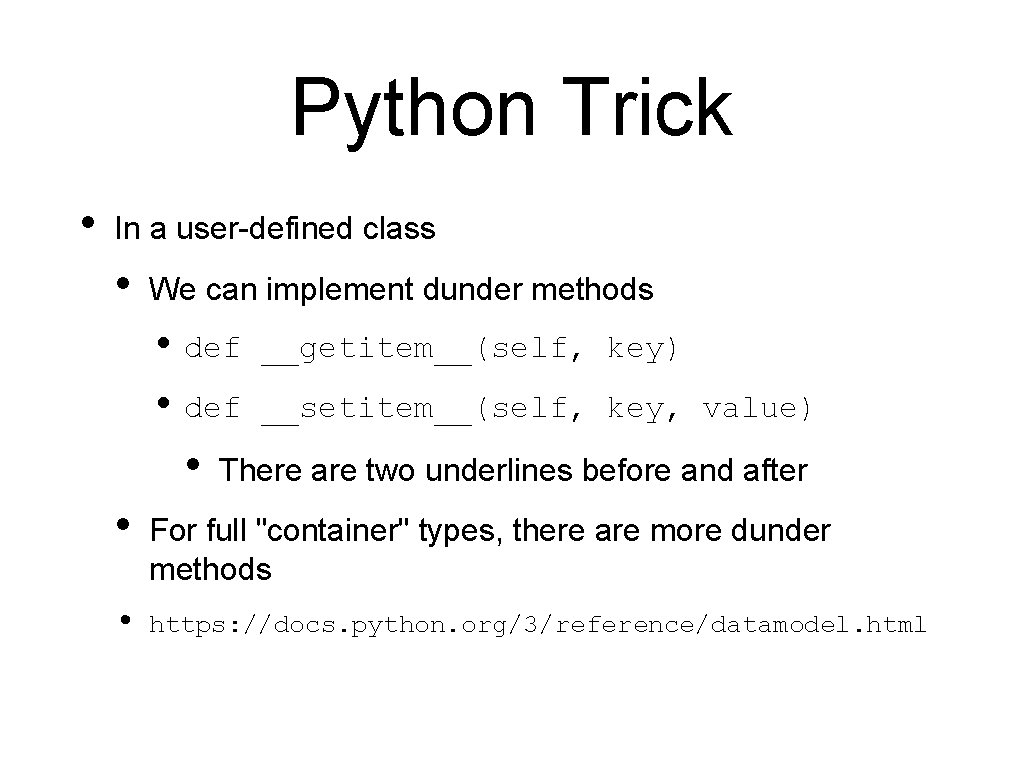Python Trick • In a user-defined class • We can implement dunder methods • def __getitem__(self, key) • def __setitem__(self, key, value) • There are two underlines before and after • • For full "container" types, there are more dunder methods https: //docs. python. org/3/reference/datamodel. htmlArray ADT • Array needs to support • • getting an item at a given location setting an item at a given location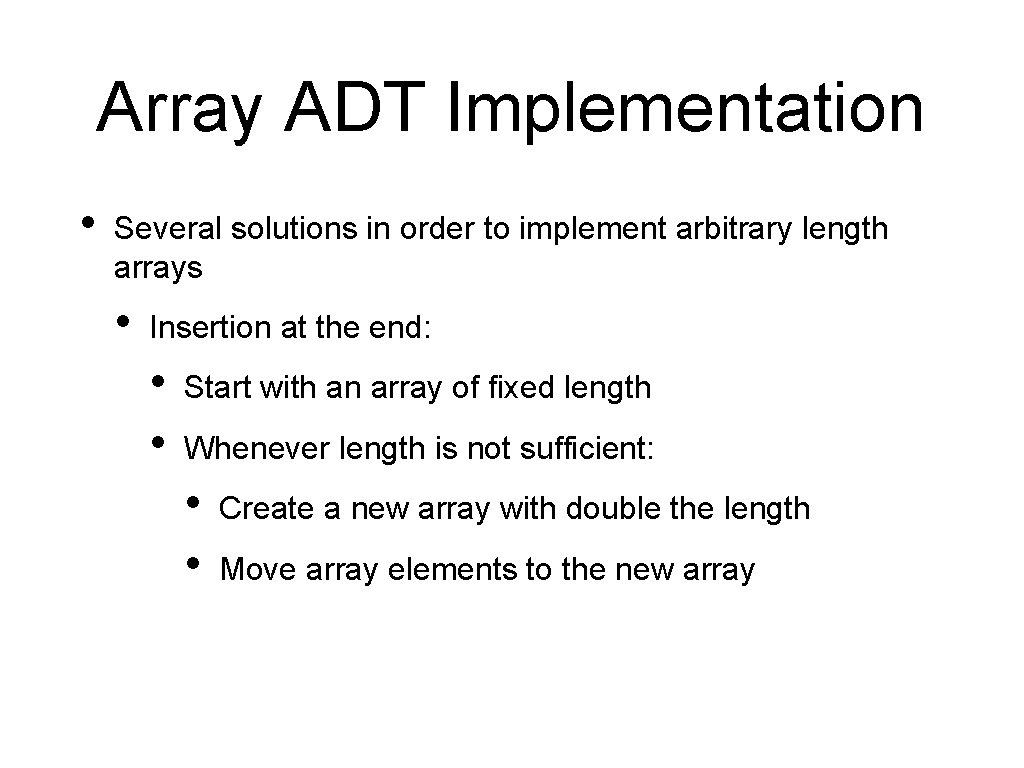Array ADT Implementation • Several solutions in order to implement arbitrary length arrays • Insertion at the end: • • Start with an array of fixed length Whenever length is not sufficient: • • Create a new array with double the length Move array elements to the new array